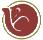# NHibernate

The object-relational mapper for .NET

# How to

This page is converted from the old nhforge.org Wiki. First published by: , Last revision by:

## Prevent NHibernate from saving default relations

Let's take a look at the following code:

public class TaxCalculator

{

public static readonly TaxCalculator NoTax = new TaxCalculator("No Tax", 0.0);

public TaxCalculator()

{

}

public TaxCalculator(String name, Double percentage)

{

this.Name = name;

this.Percentage = percentage;

}

public Decimal CalculateTax(Decimal value)

{

return value * pecentage;

}

public Guid Name { get; set; }

public String Name { get; set; }

public Decimal Percentage { get; set; }

}

public class Invoice
{

public Invoice()

: base(TaxCalculator.NoTax)

{
}

public Invoice(TaxCalculator calculator)
{
this.calculator = calculator;
}

public TaxCalculator Calculator { get; set; }
public string Description { get; set; }
public decimal Total { get; set; }

public decimal Tax
{
get { return Calculator.CalculateTax(Total); }
}
}

In this example I want to be able to save a reference to the TaxCalculator, using the following mapping:

```<class name="Invoice"> <id type="guid">     <generator class="guid"/> </id> <property name="Description"/> <property name="Total"/> <reference name="Calculator" /></class>

<class name="TaxCalculator"> <id name="Id" type="guid">     <generator class="guid"/> </id> <property name="Name"/> <property name="Percentage"/></class>```

I assume that TaxCalculators are created before I save the invoice (that is how we do it at the moment). The problem we are getting into is that we can't save a reference to TaxCalculator.NoTax. This is because the NoTax object will be created over and over again and so it will not have any Id.

The solution could be to set the Calculator property to null, but then we have to check for a null reference. But luckily it is also possible to let NHibernate set this property on null when persisting the object and set it to NoTax when loading the invoice. This is done by using Events:

public class FlushEvent : DefaultFlushEntityEventListener

{

public override void OnFlushEntity(FlushEntityEvent @event)

{

if (@event.Entity is Invoice && ((Invoice)@event.Entity).Calculator == TaxCalculator.NoTax)

((Invoice)@event.Entity).Calculator = null;

base.OnFlushEntity(@event);

}

}

{

{